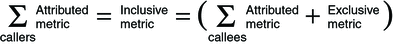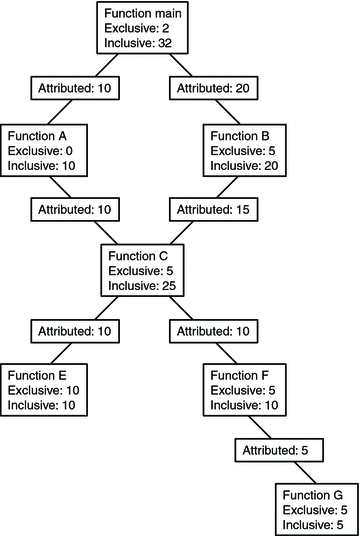Sun Studio 12: Performance Analyzer

# How Metrics Are Assigned to Program Structure

Metrics are assigned to program instructions using the call stack that is recorded with the event-specific data. If the information is available, each instruction is mapped to a line of source code and the metrics assigned to that instruction are also assigned to the line of source code. See Chapter 7, Understanding the Performance Analyzer and Its Data for a more detailed explanation of how this is done.

In addition to source code and instructions, metrics are assigned to higher level objects: functions and load objects. The call stack contains information on the sequence of function calls made to arrive at the instruction address recorded when a profile was taken. The Performance Analyzer uses the call stack to compute metrics for each function in the program. These metrics are called function-level metrics.

## Function-Level Metrics: Exclusive, Inclusive, and Attributed

The Performance Analyzer computes three types of function-level metrics: exclusive metrics, inclusive metrics and attributed metrics.

• Exclusive metrics for a function are calculated from events which occur inside the function itself: they exclude metrics coming from calls to other functions.

• Inclusive metrics are calculated from events which occur inside the function and any functions it calls: they include metrics coming from calls to other functions.

• Attributed metrics tell you how much of an inclusive metric came from calls from or to another function: they attribute metrics to another function.

For a function that only appears at the bottom of call stacks (a leaf function), the exclusive and inclusive metrics are the same.

Exclusive and inclusive metrics are also computed for load objects. Exclusive metrics for a load object are calculated by summing the function-level metrics over all functions in the load object. Inclusive metrics for load objects are calculated in the same way as for functions.

Exclusive and inclusive metrics for a function give information about all recorded paths through the function. Attributed metrics give information about particular paths through a function. They show how much of a metric came from a particular function call. The two functions involved in the call are described as a caller and a callee. For each function in the call tree:

• The attributed metrics for a function’s callers tell you how much of the function’s inclusive metric was due to calls from each caller. The attributed metrics for the callers sum to the function’s inclusive metric.

• The attributed metrics for a function’s callees tell you how much of the function’s inclusive metric came from calls to each callee. Their sum plus the function’s exclusive metric equals the function’s inclusive metric.

The relationship between the metrics can be expressed by the following equation:Comparison of attributed and inclusive metrics for the caller or the callee gives further information:

• The difference between a caller’s attributed metric and its inclusive metric tells you how much of the metric came from calls to other functions and from work in the caller itself.

• The difference between a callee’s attributed metric and its inclusive metric tells you how much of the callee’s inclusive metric came from calls to it from other functions.

To locate places where you could improve the performance of your program:

• Use exclusive metrics to locate functions that have high metric values.

• Use inclusive metrics to determine which call sequence in your program was responsible for high metric values.

• Use attributed metrics to trace a particular call sequence to the function or functions that are responsible for high metric values.

## Interpreting Attributed Metrics: An Example

Exclusive, inclusive and attributed metrics are illustrated in Figure 2–1, which contains a complete call tree. The focus is on the central function, function C.

Pseudo-code of the program is shown after the diagram.

##### Figure 2–1 Call Tree Illustrating Exclusive, Inclusive, and Attributed MetricsThe Main function calls Function A and Function B, and attributes 10 units of its inclusive metric to Function A and 20 units to function B. These are the callee attributed metrics for function Main. Their sum (10+20) added to the exclusive metric of function Main equals the inclusive metric of function main (32).

Function A spends all of its time in the call to function C, so it has 0 units of exclusive metrics.

Function C is called by two functions: function A and function B, and attributes 10 units of its inclusive metric to function A and 15 units to function B. These are the caller attributed metrics. Their sum (10+15) equals the inclusive metric of function C (25)

The caller attributed metric is equal to the difference between the inclusive and exclusive metrics for function A and B, which means they each call only function C. (In fact, the functions might call other functions but the time is so small that it does not appear in the experiment.)

Function C calls two functions, function E and function F, and attributes 10 units of its inclusive metric to function E and 10 units to function F. These are the callee attributed metrics. Their sum (10+10) added to the exclusive metric of function C (5) equals the inclusive metric of function C (25).

The callee attributed metric and the callee inclusive metric are the same for function E and for function F. This means that both function E and function F are only called by function C. The exclusive metric and the inclusive metric are the same for function E but different for function F. This is because function F calls another function, Function G, but function E does not.

Pseudo-code for this program is shown below.

```    main() {
A();
/Do 2 units of work;/
B();
}

A() {
C(10);
}

B() {
C(7.5);
/Do 5 units of work;/
C(7.5);
}

C(arg) {
/Do a total of "arg" units of work, with 20% done in C itself,
40% done by calling E, and 40% done by calling F./
}```

## How Recursion Affects Function-Level Metrics

Recursive function calls, whether direct or indirect, complicate the calculation of metrics. The Performance Analyzer displays metrics for a function as a whole, not for each invocation of a function: the metrics for a series of recursive calls must therefore be compressed into a single metric. This does not affect exclusive metrics, which are calculated from the function at the bottom of the call stack (the leaf function), but it does affect inclusive and attributed metrics.

Inclusive metrics are computed by adding the metric for the event to the inclusive metric of the functions in the call stack. To ensure that the metric is not counted multiple times in a recursive call stack, the metric for the event is only added to the inclusive metric for each unique function.

Attributed metrics are computed from inclusive metrics. In the simplest case of recursion, a recursive function has two callers: itself and another function (the initiating function). If all the work is done in the final call, the inclusive metric for the recursive function is attributed to itself and not to the initiating function. This attribution occurs because the inclusive metric for all the higher invocations of the recursive function is regarded as zero to avoid multiple counting of the metric. The initiating function, however, correctly attributes to the recursive function as a callee the portion of its inclusive metric due to the recursive call.